# Determine your size for bathroom sinks

Flex P-trap can be used in all situations under sink as a p-trap and as a connecting tube between parts. Because of this, you have to determine the size (length of tube) or cut the tube yourself. If you are looking from outside inside of cabinet, you have the right length of Flex P-trap noted with R and left length notated with L . The tolerance for measurement is ±0.5 inches.

1. First, you have to choose one position from Fig.33 - Fig.46
2. Second, you have to measure dimension JK or A, H, G.
3. Third, you choose length R and L from bottom measurement.

NOTE: Fig.34B, (3/4 CP-trap) Fig.33D, (3/4 Flex p-trap) it is not recommended because it could be siphoning if the house doesn’t have good vent. The 3/4 Flex p-trap it is a unique solution for bathroom sink with shelf A-H=2.0. The 3/4 CP-trap is very simple for a bathroom sink with shelf A-H=4.5.

Flex p-trap

Full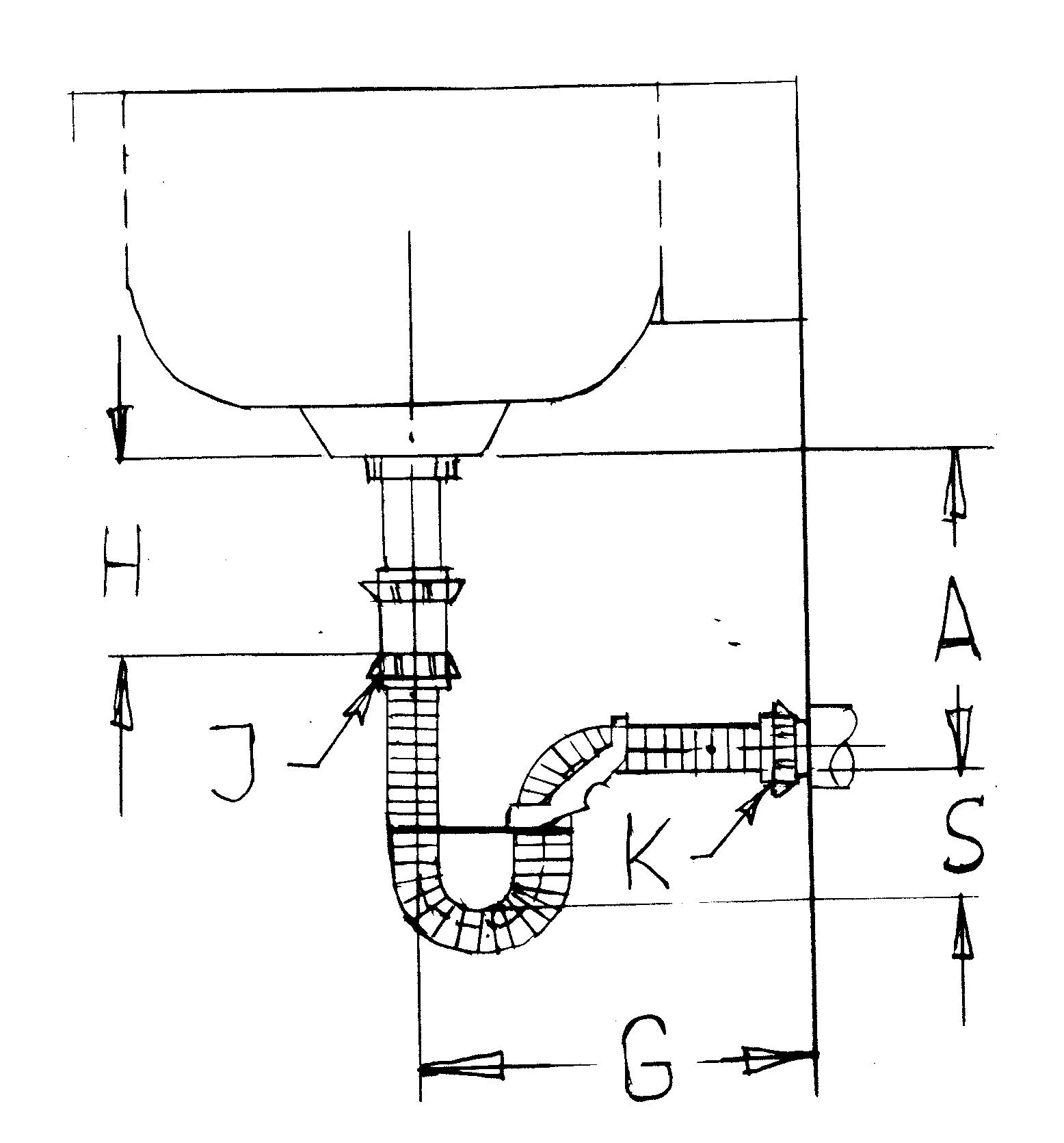Fig.33 A (video)

G=8—10 inches

H=length of existing tailpiece

A=position of drain

L=length of flex p-trap

8 inches---length of U shape (2+2+4) for 2 inches water seal

2 inches—length of coupling.

Minimum water seal of p-trap with adaptor is 3.0 inches

E=0--5 inches—eccentricity (left or right)

S—water seal

For Smin=2 inches for p-trap without adaptor and E=0

L=G-4+2+8+(A-H)=G+(A-H)+6

G=10           L=G+(A-H)+6=10+( A-H)+6   L=A-H+16

For A-H=0   Lmin= 16 inches

For A-H=2   L= 18 inches

For A-H=4   L= 20 inches

For A-H=6   Lmax= 22 inches

For E=0-5  A-H=0

L=D+(A-H)+6=D+6      D2=E2+G2

A-H=0    G=10   D2=100+25    D=11.2

L=D+6=11.2+6=17.2=18

Flex p-trap with 18 inches length can be installed 5 inches right, left with 2 inches water seal and A=H. If drain is 2 inches down, A-H=2 you need L= D+2+6=20 inches length (2 inches water seal and 5 inches left or right). For certain positions of drain A-H=2  Flex p-trap with L=22 inches can be installed 5 inches right, left and 3 inches water seal.

For A-H=0 G=10

L=16 inches—Flex p-trap, 2 inches water seal E=0 inches left, right

L=18 inches—Flex p-trap, 2-3 inches water seal E=2-0 inches left, right

L=20 inches—Flex p-trap, 3-4 inches water seal E=3-0 inches left, right

L=22 inches—Flex p-trap, 4-5 inches water seal E=4-0 inches left, right

Water seal S=2-5  E=0  G=10  A=H

If you add (T) 2 inches to the length of p-trap, the water seal will increase with 1 inches.

T=(S-2)2      T+Lmin=L   (S-2)2+16=L    2S-4+16=L   S=L/2-12/2   S=L/2-6

For  T=0              L=16      E=0   0=2S-4  S=2”

T=2              L=18”     E=0   S=18/2-6=3”

T=4              L=20      E=0   S=20/2-6=4”

T=6              L=22      E=0   S=22/2-6=5”

If you move the end of the p-trap right, left or down the water seal will decrease, but if you move it up the water seal will increase.

The adaptor makes with the corrugated tube a simple mechanism with two degree of freedom and control a curvature of corrugated tube on one arm of U-shape, and slide along a length of ouside surface of corrugated tube and rotate around the axis to adjust the p-trap in space. The adapter control the outside surface of corrugated tube in three spots: the ends of adaptor and in midle of adaptor (interior surface) some as three points on the curve. The adaptor can be replaced with plastic (rubber) cord and the flex p-trap will work like an adjustable one piece p-trap, where increasing the length of the p-trap will decrease the water seal. The 16 inch p-trap is only recommended when you don't have enough room under the sink or if the cabinet has a shelfThe adaptor can be optional for the full Flex p-trap, but for the S-trap and SP-trap it's absolutely necessary. The adaptor can be used over 16 inches length of p-trap.

Measurement

Our converter formula was specially designed to make you figure out your job a lot easier. Measured distance JK in space and length of Flex p-trap will be:

L=JK+10”

J—end of tailpiece

K—bottom of drain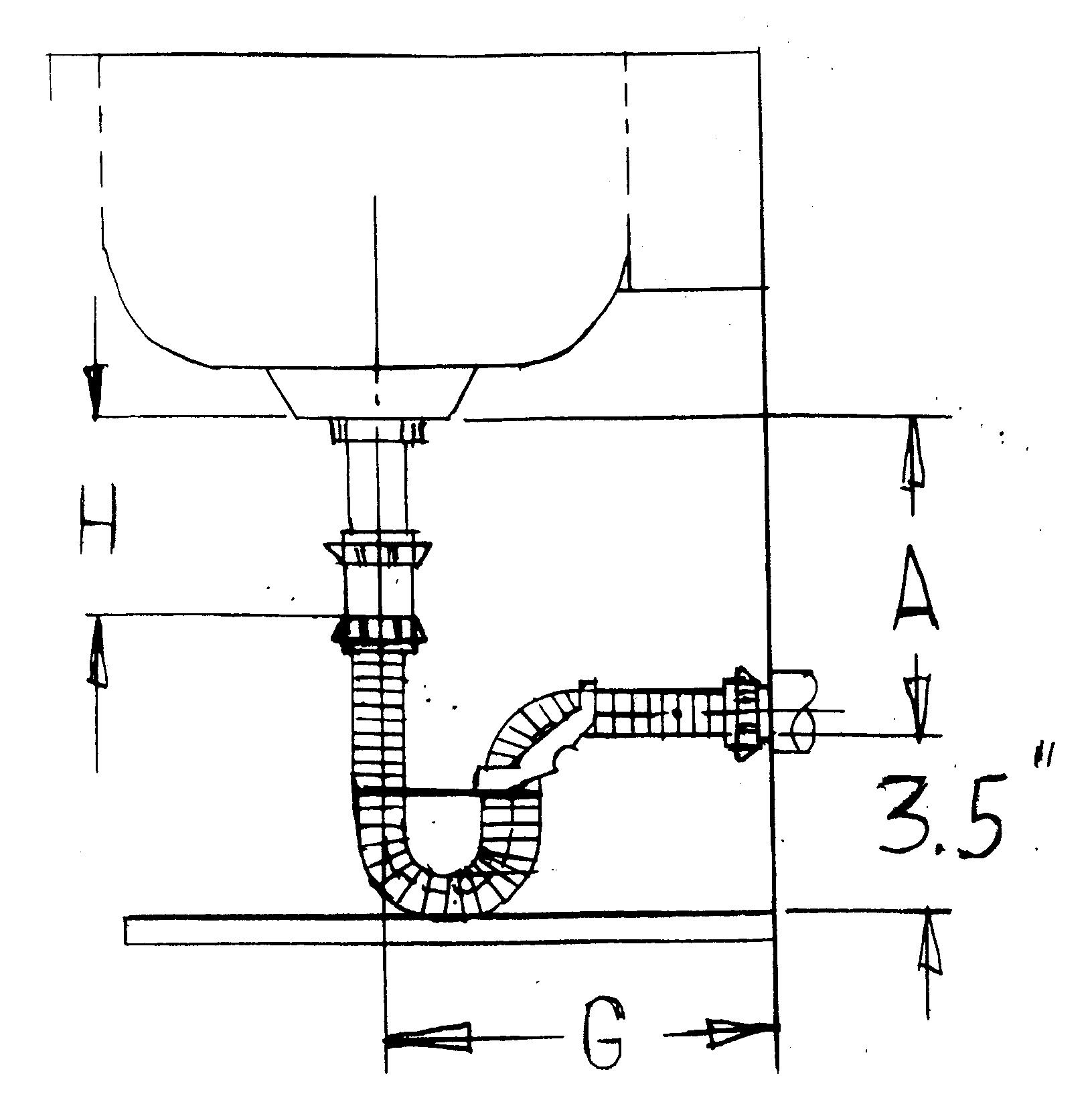FIG.33 B

If the bathroom cabinet has a shelf, the minimum distance from drain to shelf is 3.5 inches for 2.0 inches water seal, similar as the regular p-trap, classic installation.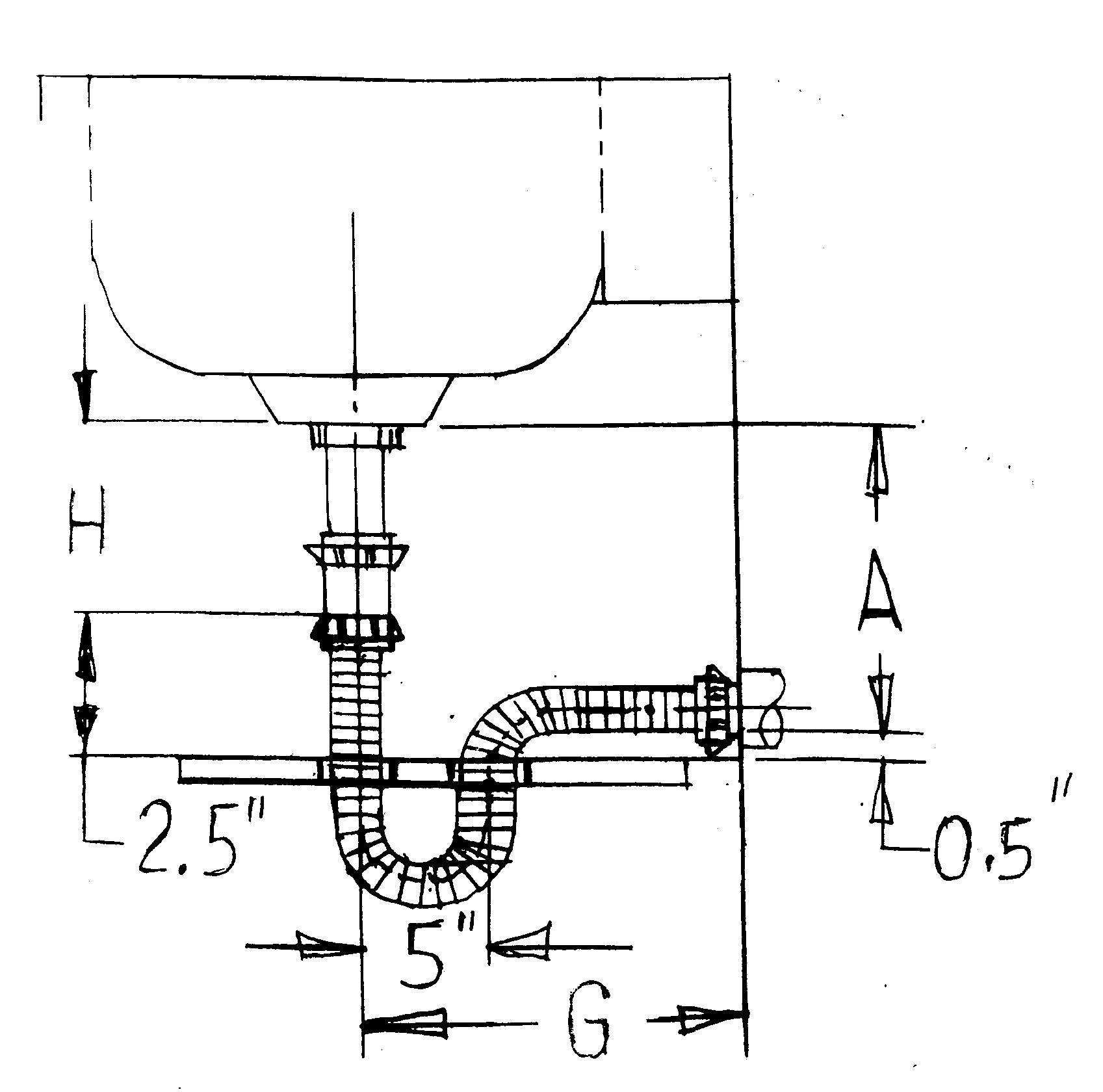FIG.33C

If the distance from the drain to shelf is minimum 0.5 inches and the distance from tailpiece of sink to shelf is minimum 2.5 inches you have to drill two holes 1.75 inches diameter in the shelf, disconnect the last nut from Flex p-trap and install the tube in the holes. See the Fig.33C; Second option is Fig.33D.

3/4Flex p-trap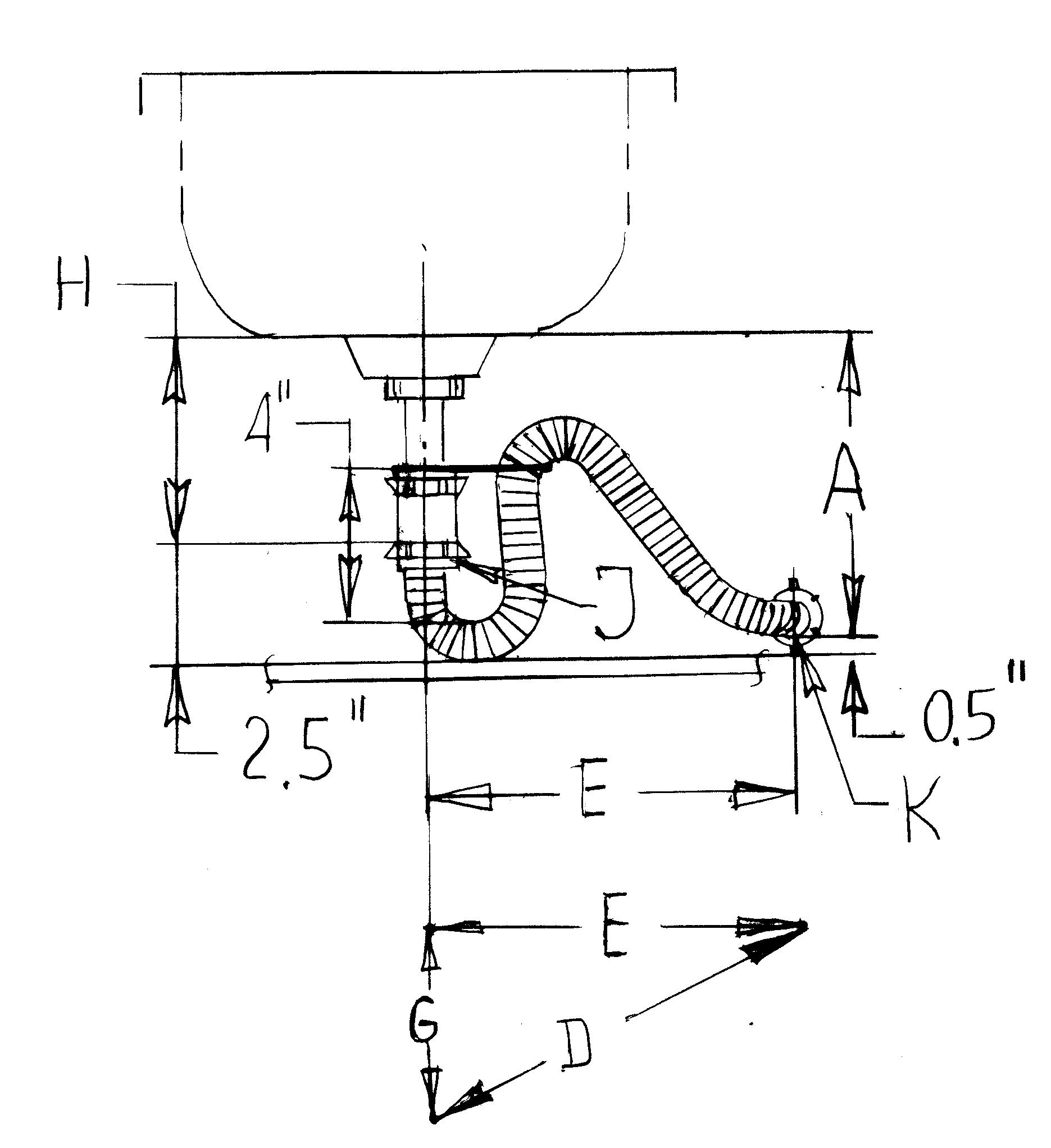Fig.33D (video)

After remodeling the bathroom, the sink can be moved right or left with distance E=5-10 inches

If you have 2.5 inches from bottom of the tailpiece to shelf and 0.5 inches from drain to shelf you can install the Flex p-trap in four steps:

1. Disconnect the coupling from Flex p-trap and installing it on tailpiece.

2. Connect the end of tubing with a drain with last nut.

3. Push the other end of tubing in tailpiece and connect the coupling with first nut.

4. Installing the rubber cord and plastic cord for hold the shape of p-trap.

Lmax=A-H+2+2.5+4+1.5+4+D-4=A-H+D+10

Lmax=A-H+D+10

For A-H=2 inches

Lmax=2+D+10=D+12

Lmax=D+12

For G=10  E=10  A-H=2

D2=E2+G2

D2=100+100    D=14

Lmax=D+12=14+12=26

For G=10  E=7  A-H=2

D2=100+49    D=12

Lmax=D+12=12+12=24

S=4 water seal

Third option is Fig.34B

Measurement

Our converter formula was specially designed to make you figure out your job a lot easier. Measure distance JK in space and length of Flex p-trap will be:

L=JK+10”

J—end of tailpiece

K—bottom of drain

CP-trap

Connector tube with p-trap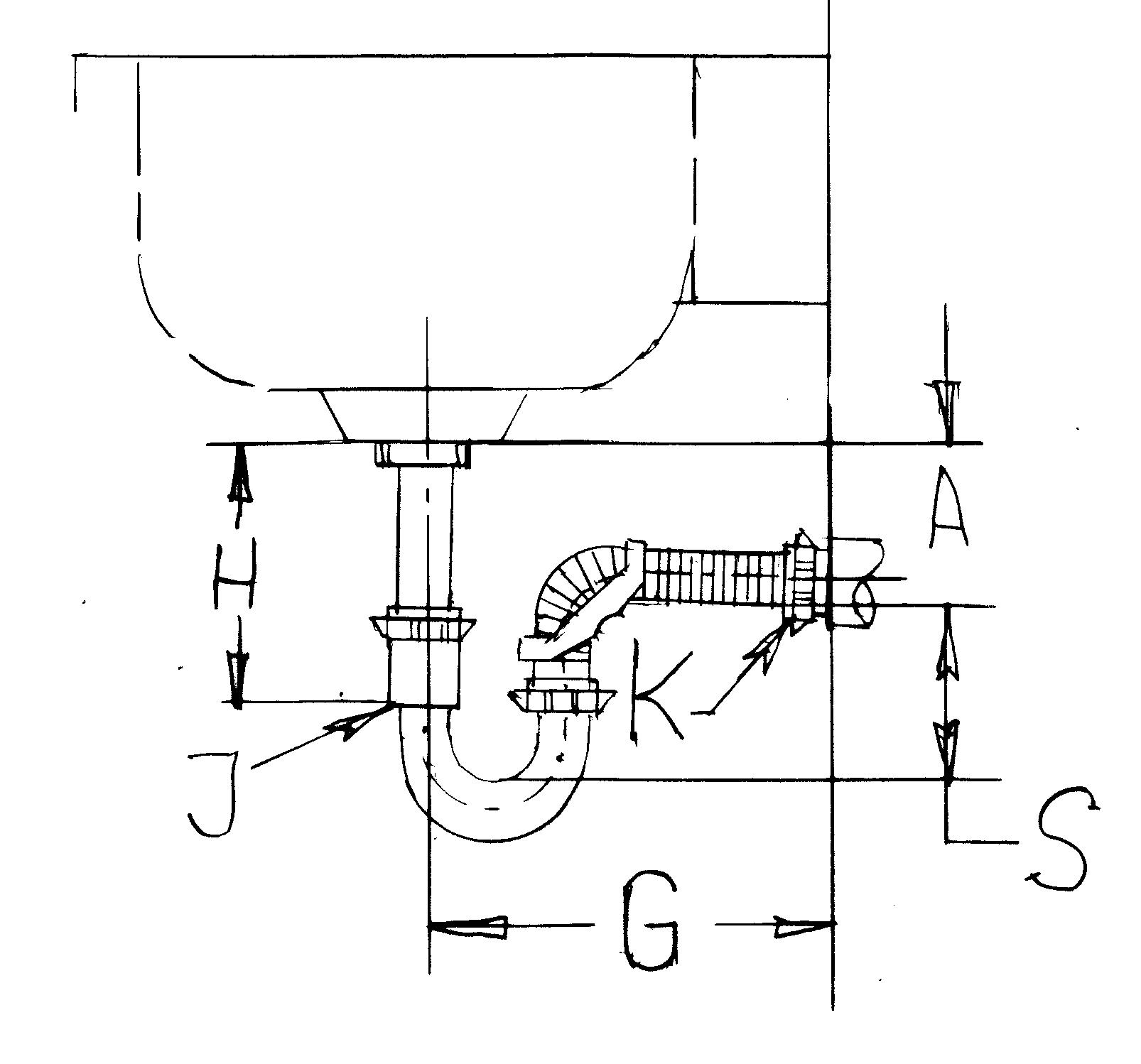Fig.34A  (video)

G=4—10 inches E=0-4

8 inches—length of U shape of regular p-trap

E--eccentricity

H—length of tailpiece

A—position of drain

H-A=2 inches, (for assembling the adaptor with tube we need 2 inches)

For G=10 inches and E=0

L = G-4+2+8=G+6

Lmin=G+6=10+6=16 inches

For G=4 inches, the p-trap will be rotated around tailpiece 180 degree

L=G+4+2+8=G+14

L= G+14=4+14=18 and E=0

Rotate the p-trap around tailpiece G will be D and drain can be moved right or left E=0-4

L=D-4+2+8=D+6

L=D+6

For G=10    E=4      D2=E2+G2

D2=100+16    D=10.77=11

L=D+6=6+11=17

Rotate p-trap around tailpiece 180 degree and E=4

L=D+4+2+8=D+14

L=D+14=11+14=25   Lmax=22   or   Lmax=24

The p-trap with 22 inches length can’t be rotated full 180 degree but will cover G=4-10 and E=0-4 inches.

For H-A=2  G=10

L=16 inches—Flex p-trap, 3 inches water seal E=0 inches left, right

L=18 inches—Flex p-trap, 3-4 inches water seal E=2-0 inches left, right

L=20 inches—Flex p-trap, 4-5 inches water seal E=3-0 inches left, right

L=22 inches—Flex p-trap, 5-6 inches water seal E=4-0 inches left, right

Water seal S=3-6  E=0  G=10

Smin=3 water seal

Lmin=G+6=10+6=16 inches

If you add (T) 2 inches to the length of p-trap, the water seal will increase with 1 inches

T=(S-3)2      T+Lmin=L   (S-3)2+16=L    2S-6+16=L   S=L/2-10/2   S=L/2-5

For T=0               L=16      E=0   0=2S-6  S=3”

T=2              L=18”     E=0   S=18/2-5=4”

T=4              L=20      E=0   S=20/2-5=5”

T=6              L=22      E=0   S=22/2-5=6

If H=constant,  G=10  E=0 and Smin=3 the drain can be lift up with 4 inches for Flex p-trap with L=20 inches and 6 inches for Flex p-trap L=22 inches.

The p-trap with 22 inches length can’t be rotated full 180 degree but will cover G=4-10 and E=0-4 inches. Adaptor can be optional. The regular p-trap will make with the corrugated tube a Dwell linkage mechanism with one flexible element. The length of p-trap it is adjustable by rotating the regular p-trap around the axis of its taipiece.

Measurement

Our converter formula was specially designed to make you figure out your job a lot easier. Measure distance JK in space and length of Flex p-trap will be:

L=JK+8”

J—end of tailpiece

K—bottom of drain

3/4CP-trap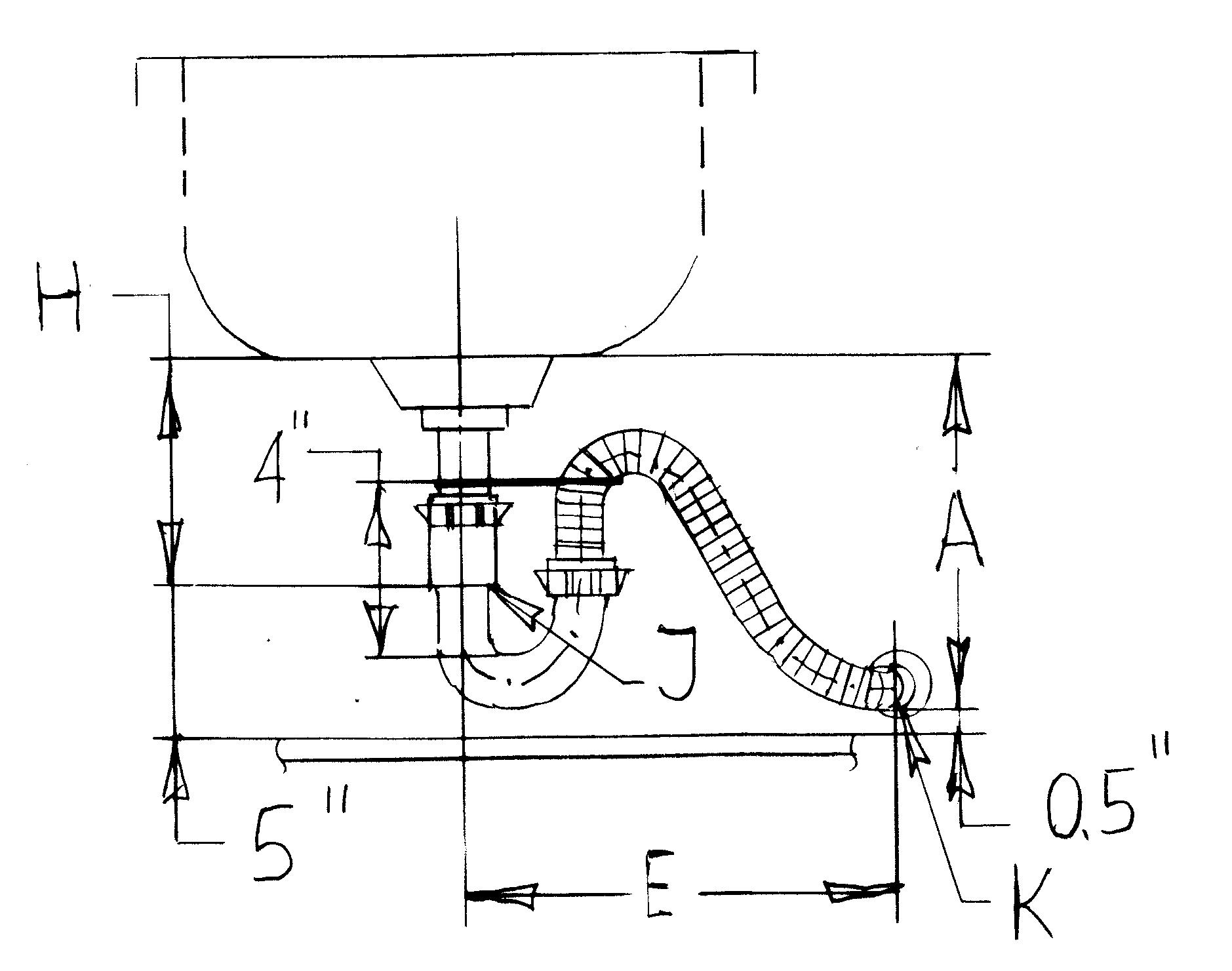Fig.34B (video)

If you have 2.5 inches from the bottom of the tailpiece to shelf and 0.5 inches from the drain to shelf you can use CP-trap. First you have to cut 2.5-2.0 inches from the end of the tailpiece. The height of the p-trap is 4.5 inches 5-2.5=2.5 inches or 4.5-2.5=2.0 inches. Install the regular p-trap with the tailpiece, and the end of tubing with a drain. Last step is to install the rubber cord.

S=4 water seal

L=8+2+D-4=D+6   For 180 degree rotated

Lmax=4+D+6=D+10

For A-H=4.5 and E=0-10

G=10    E=10      D2=E2+G2

D2=100+100    D=14

Lmax=D+10=14+10=24

Measurement

Our converter formula was specially designed to make you figure out your job a lot easier. Measure distance JK in space and length of Flex p-trap will be:

L=JK+10”

J—end of tailpiece

K—bottom of drain

3/4SP-trap

Fig.34C (video)

If you have room, you can add one adapter Fig.34C. Standard S-trap isn’t approved in some states because sometime siphoning of standard S-trap. To stop the siphoning, the S-trap needs 3 inches (2Dia) in horizontal position or to increase the deep seal of the S-trap.

L=D-4+2+8=D+6   For 180 degree rotated

Lmax=4+D+6=D+10

Measurement

Our converter formula was specially designed to make you figure out your job a lot easier. Measure distance JK in space and length of Flex p-trap will be:

L=JK+12”

J—end of tailpiece

K—bottom of drain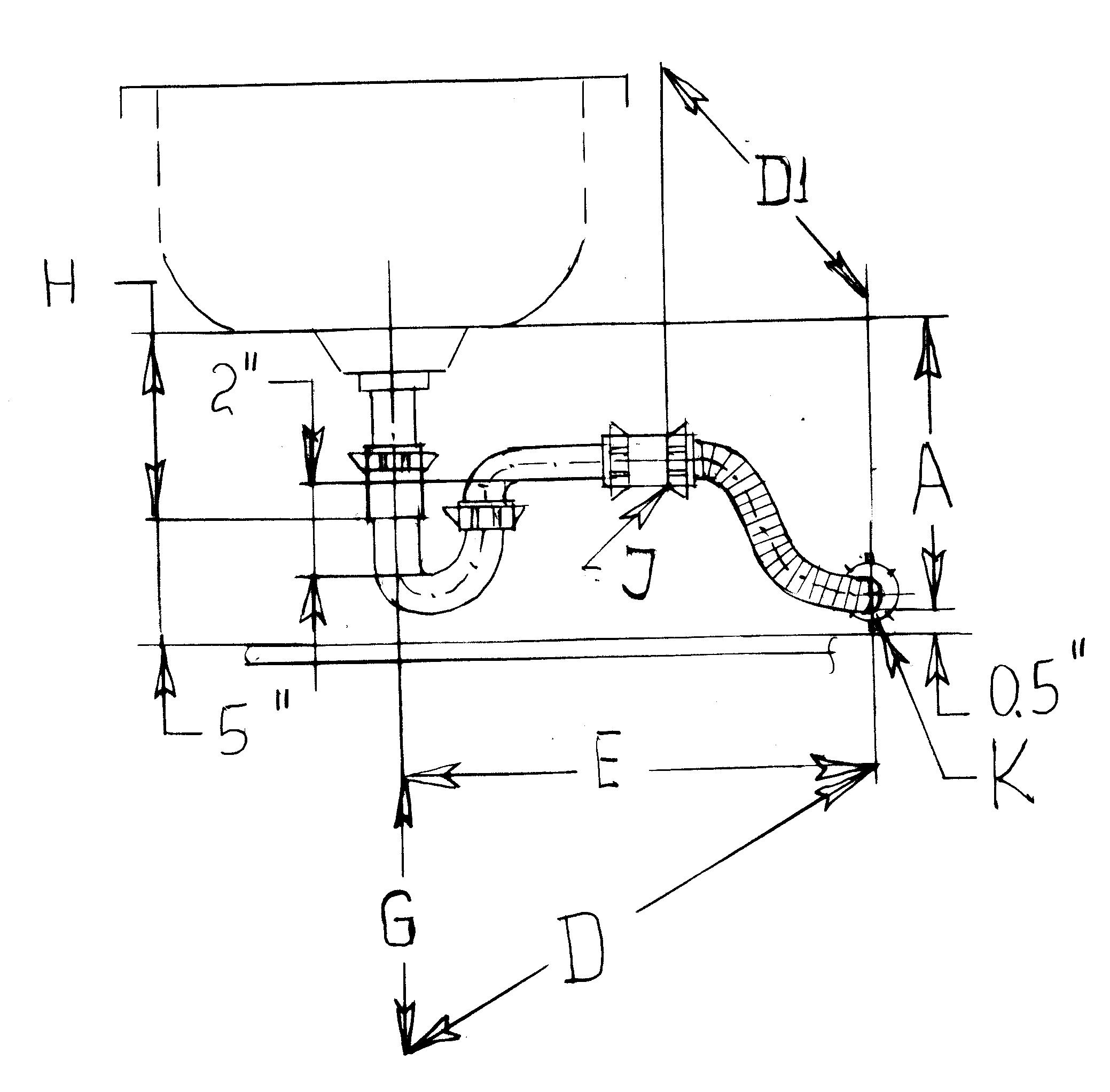Fig.34D (video)

After remodeling the bathroom, the sink can be moved to the right or left with distance E=0-18, (5 inches under the tailpiece A-H=5) and drain 0.5 inches above shelf, you can use Fig.34D.

The regular p-trap makes with the corrugated tube a four-bar mechanism with one flexible element. The length of the p-trap it is adjustable by rotating the regular p-trap around the axis of the taipiece.

Measurement

Our converter formula was specially designed to make you figure out your job a lot easier. Adjust in space the regular p-trap how you want it to be and measure distance JK.

The length of Flex p-trap will be:

L=JK+5"

J—end of horizontal arm

K—bottom of drain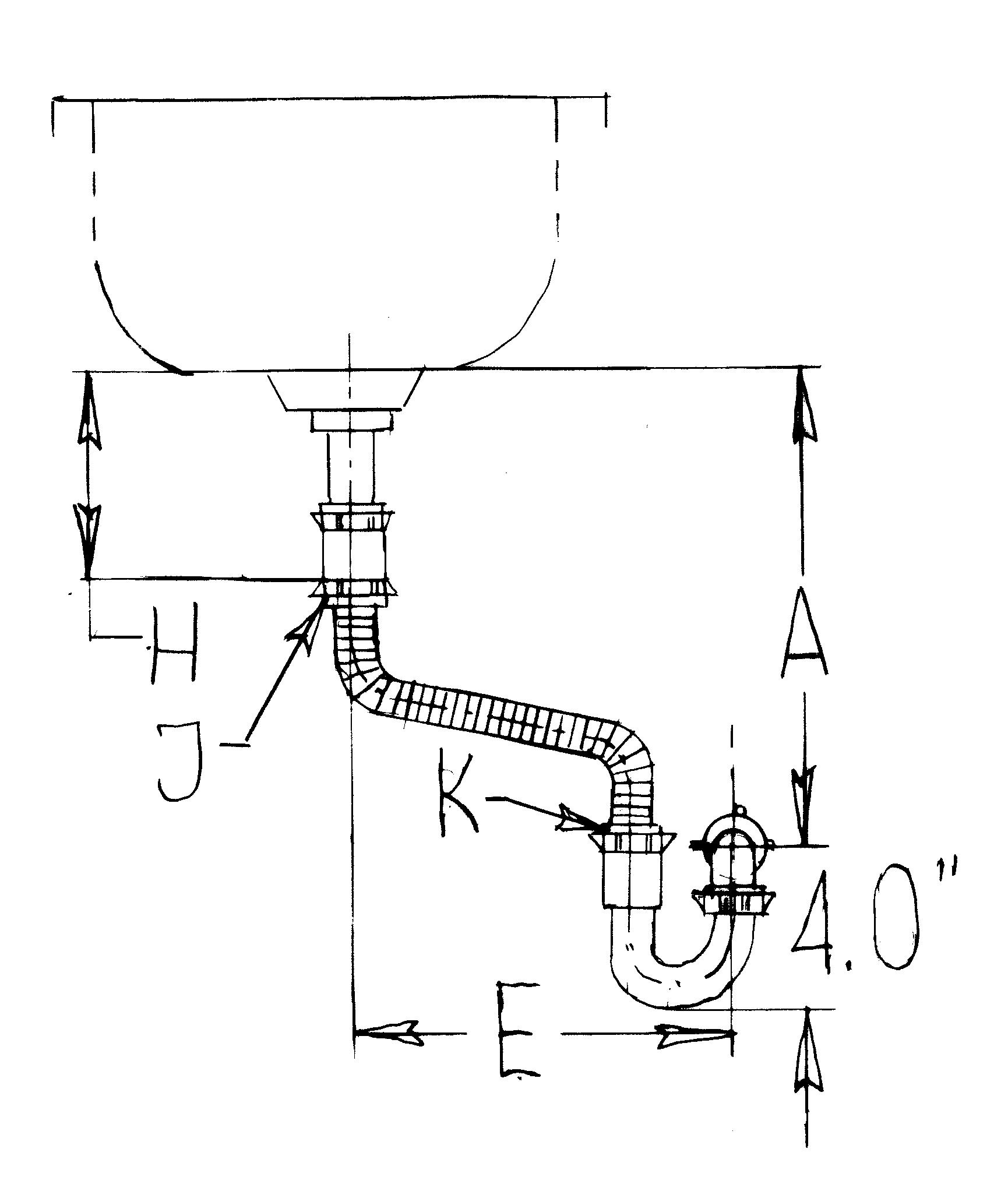Fig.34E (video)

After remodeling the bathroom, the sink can be moved to the right or left with distance E=0--12 and drain 4.0-4.5 inches above shelf (floor), in this situation you can use Fig.34E.

Measurement

Our converter formula was specially designed to make you figure out your job a lot easier. Adjust in space the regular p-trap how you want it to be and measure the distance JK.

The length of Flex p-trap will be:

L=JK+5”

J—end of tailpiece

K—end of p-trap

SP-trap

S-trap with p-trap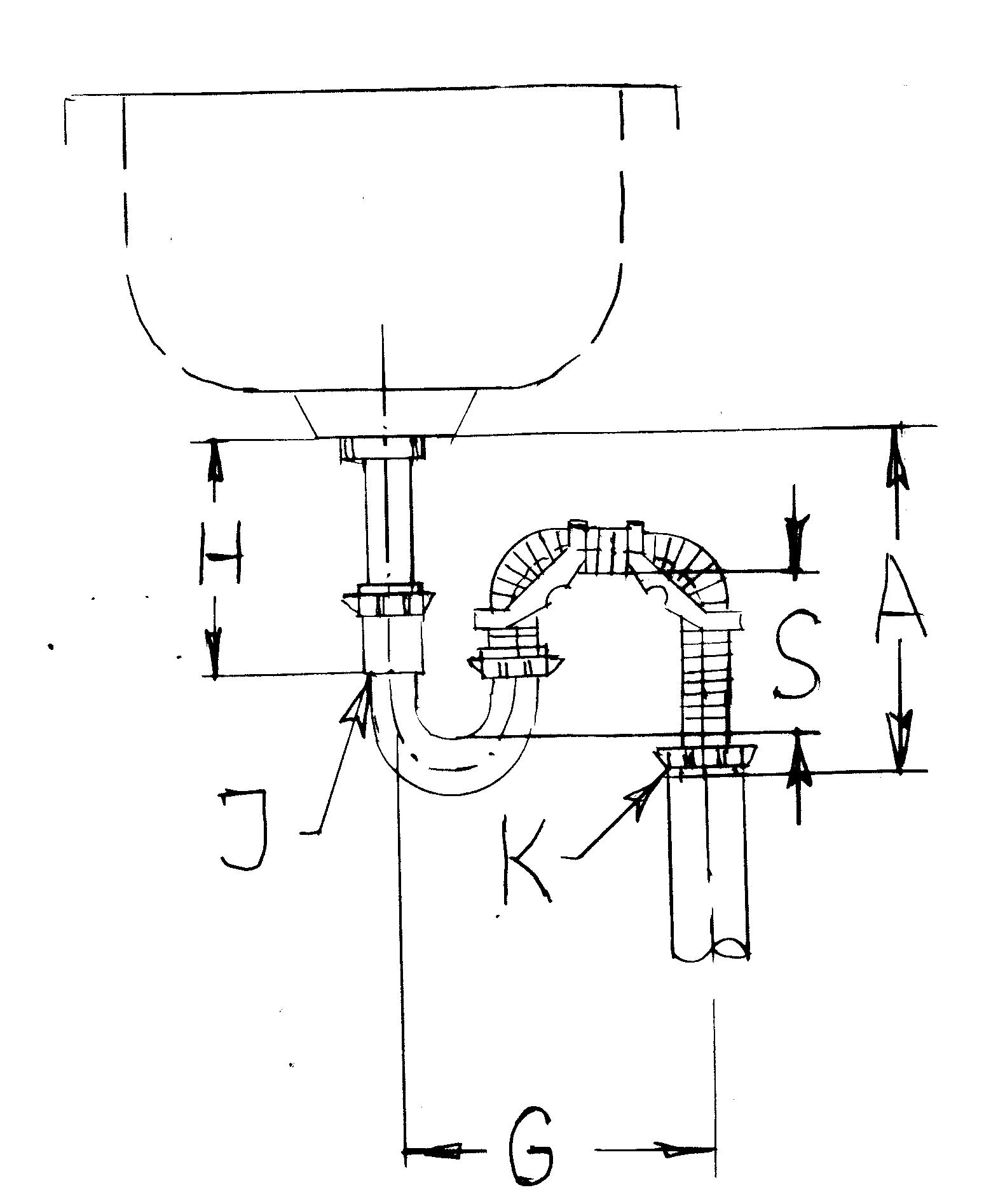Fig. 35 (video)

G=4--14 inches

Ex= extension (distance between two adaptors)

For Ex=0  A-H=0    Gmin=8

Ex=G-8   over Gmin=8 inches you need to add extension

Lmin=16

If you add (T) 2 inches to the length of p-trap, the water seal will increase with 1 inch

If H=constant,  G=14  E=0 and Smin=3  the drain can be lowered up to 8 inches for Flex p-trap with L=24 inches

In order to eliminate the siphoning, the minimum extension has to be Ex=2Dia=2.5

Measurement

Our converter formula was specially designed to make you figure out your job a lot easier. Measure distance JK in space and length of Flex p-trap will be:

L=JK+16”  J—end of tailpiece  K—bottom of drain

S-trap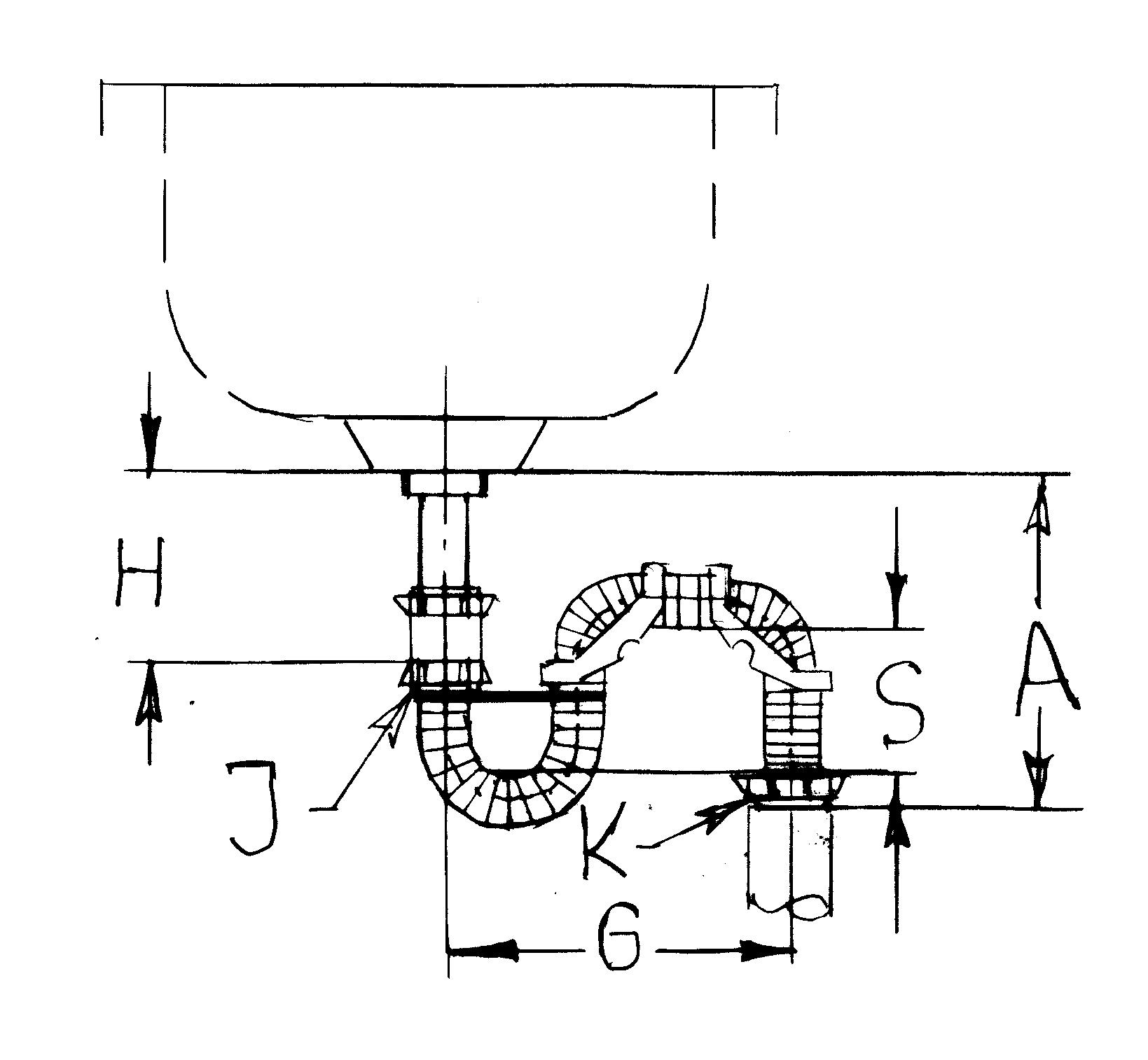Fig.36 (video 35)

G=8--16 inches

Ex= extension (distance between two adapters)

8 inches -- length of U shape (2+2+4) bottom of adapter

2 inches -- length of coupling

For Ex=0  A-H=0  Gmin=8

Ex=G-8   over Gmin=8 inches you need to add extension

Rotate p-trap around the tailpiece G will be D and the drain can be moved right or left with E.

In order to eliminate the siphoning, the minimum extension has to be Ex=2Dia=2.5

Measurement

Our converter formula was specially designed to make you figure out your job a lot easier. Measure distance JK in space and length of Flex p-trap will be:

L=JK+18”

J—end of tailpiece

K—bottom of drain

Two sinks one drain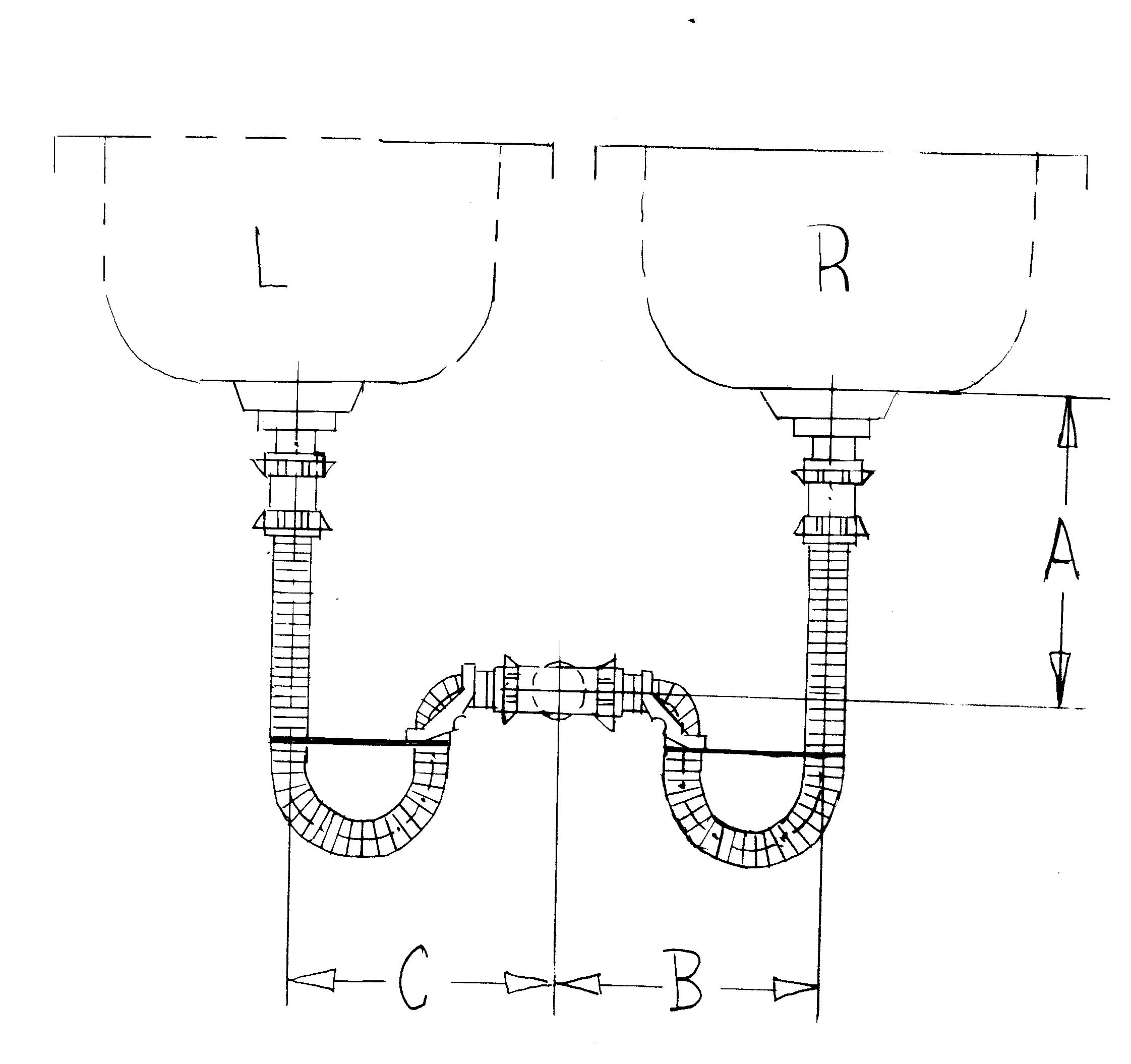Fig. 46

For A=maximum